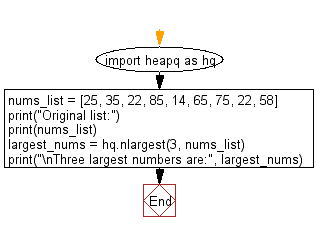﻿ Python heap queue algorithm: Find the three smallest integers from a given list of numbers using Heap queue algorithm - w3resource

# Python: Find the three smallest integers from a given list of numbers using Heap queue algorithm

## Python heap queue algorithm: Exercise-2 with Solution

Write a Python program to find the three smallest integers from a given list of numbers using the heap queue algorithm.

Sample Solution:

Python Code:

``````import heapq as hq
nums_list = [25, 35, 22, 85, 14, 65, 75, 22, 58]
print("Original list:")
print(nums_list)
# Find three smallest values
largest_nums = hq.nsmallest(3, nums_list)
print("\nThree smallest numbers are:", largest_nums)
```
```

Sample Output:

```Original list:
[25, 35, 22, 85, 14, 65, 75, 22, 58]

Three smallest numbers are: [14, 22, 22]
```

Flowchart:Python Code Editor:

Have another way to solve this solution? Contribute your code (and comments) through Disqus.

What is the difficulty level of this exercise?

Test your Programming skills with w3resource's quiz.

﻿